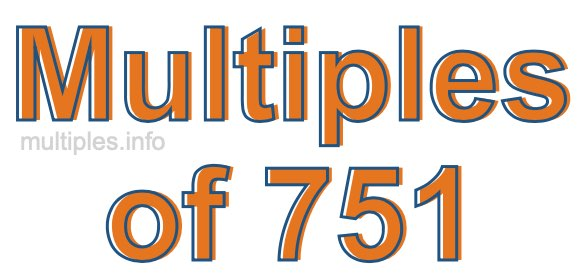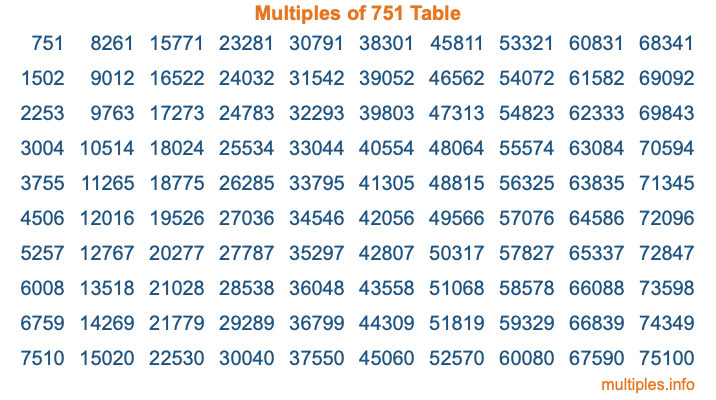Multiples of 751Welcome to the Multiples of 751 page. Here we will first teach you everything you will ever need to know about the multiples of 751, and then give you a study guide summary of everything we taught you to make sure you remember it all. Use this page to look up facts and learn information about the multiples of 751. This page will make you a multiples of seven hundred fifty-one expert!

Definition of Multiples of 751
Multiples of 751 are all the numbers that when divided by 751 equal an integer. Each of the multiples of 751 are called a multiple. A multiple of 751 is created by multiplying 751 by an integer.

Therefore, to create a list of multiples of 751, you start with 1 multiplied by 751, then 2 multiplied by 751, then 3 multiplied by 751, and so on for as long as you want. Thus, the list of the first five multiples of 751 is 751, 1502, 2253, 3004, and 3755. To see a larger list of multiples of 751, see the printable image of Multiples of 751 further down on this page. We also have a category where you can choose any nth multiple of 751.

Multiples of 751 Checker
The Multiples of 751 Checker below checks to see if any number of your choice is a multiple of 751. In other words, it checks to see if there is any number (integer) that when multiplied by 751 will equal your number. To do that, we divide your number by 751. If the the quotient is an integer, then your number is a multiple of 751.

Is  a multiple of 751?

Least Common Multiple of 751 and ...
A Least Common Multiple (LCM) is the lowest multiple that two or more numbers have in common. This is also called the smallest common multiple or lowest common multiple and is useful to know when you are adding our subtracting fractions. Enter one or more numbers below (751 is already entered) to find the LCM.

Check out our LCM Calculator if you need more details about the Least Common Multiple or if you need the LCM for different numbers for adding and subtraction fractions.

nth Multiple of 751
As we stated above, 751 is the first multiple of 751, 1502 is the second multiple of 751, 2253 is the third multiple of 751, and so on. Enter a number below to find the nth multiple of 751.

th multiple of 751

Multiples of 751 vs Factors of 751
751 is a multiple of 751 and a factor of 751, but that is where the similarities end. All postive multiples of 751 are 751 or greater than 751. All positive factors of 751 are 751 or less than 751.

Below is the beginning list of multiples of 751 and the factors of 751 so you can compare:

Multiples of 751: 751, 1502, 2253, 3004, 3755, etc.

Factors of 751: 1, 751

As you can see, the multiples of 751 are all the numbers that you can divide by 751 to get a whole number. The factors of 751, on the other hand, are all the whole numbers that you can multiply by another whole number to get 751.

It's also interesting to note that if a number (x) is a factor of 751, then 751 will also be a multiple of that number (x).

Multiples of 751 vs Divisors of 751
The divisors of 751 are all the integers that 751 can be divided by evenly. Below is a list of the divisors of 751.

Divisors of 751: 1, 751

The interesting thing to note here is that if you take any multiple of 751 and divide it by a divisor of 751, you will see that the quotient is an integer.

Multiples of 751 Table
Below is an image of the first 100 multiples of 751 in a table. The table is in chronological order, column by column. The first column has the first ten multiples of 751, the second column has the next ten multiples of 751, and so on.The Multiples of 751 Table is also referred to as the 751 Times Table or Times Table of 751. You are welcome to print out our table for your studies.

Negative Multiples of 751
Although not often discussed or needed in math, it is worth mentioning that you can make a list of negative multiples of 751 by multiplying 751 by -1, then by -2, then by -3, and so on, to get the following list of negative multiples of 751:

-751, -1502, -2253, -3004, -3755, etc.

Multiples of 751 Summary
Below is a summary of important Multiples of 751 facts that we have discussed on this page. To retain the knowledge on this page, we recommend that you read through the summary and explain to yourself or a study partner why they hold true.

There are an infinite number of multiples of 751.

A multiple of 751 divided by 751 will equal a whole number.

751 divided by a factor of 751 equals a divisor of 751.

The nth multiple of 751 is n times 751.

The largest factor of 751 is equal to the first positive multiple of 751.

751 is a multiple of every factor of 751.

751 is a multiple of 751.

A multiple of 751 divided by a divisor of 751 equals an integer.

751 divided by a divisor of 751 equals a factor of 751.

Any integer times 751 will equal a multiple of 751.

Multiples of a Number
Here you can get the multiples of another number, all with the same attention to detail as we did for multiples of 751 on this page.

Multiples of
Multiples of 752
Did you find our page about multiples of seven hundred fifty-one educational? Do you want more knowledge? Check out the multiples of the next number on our list!